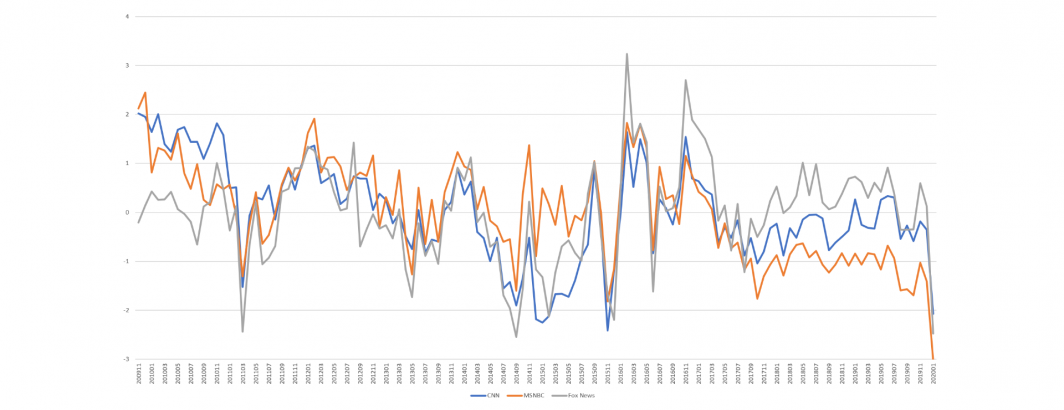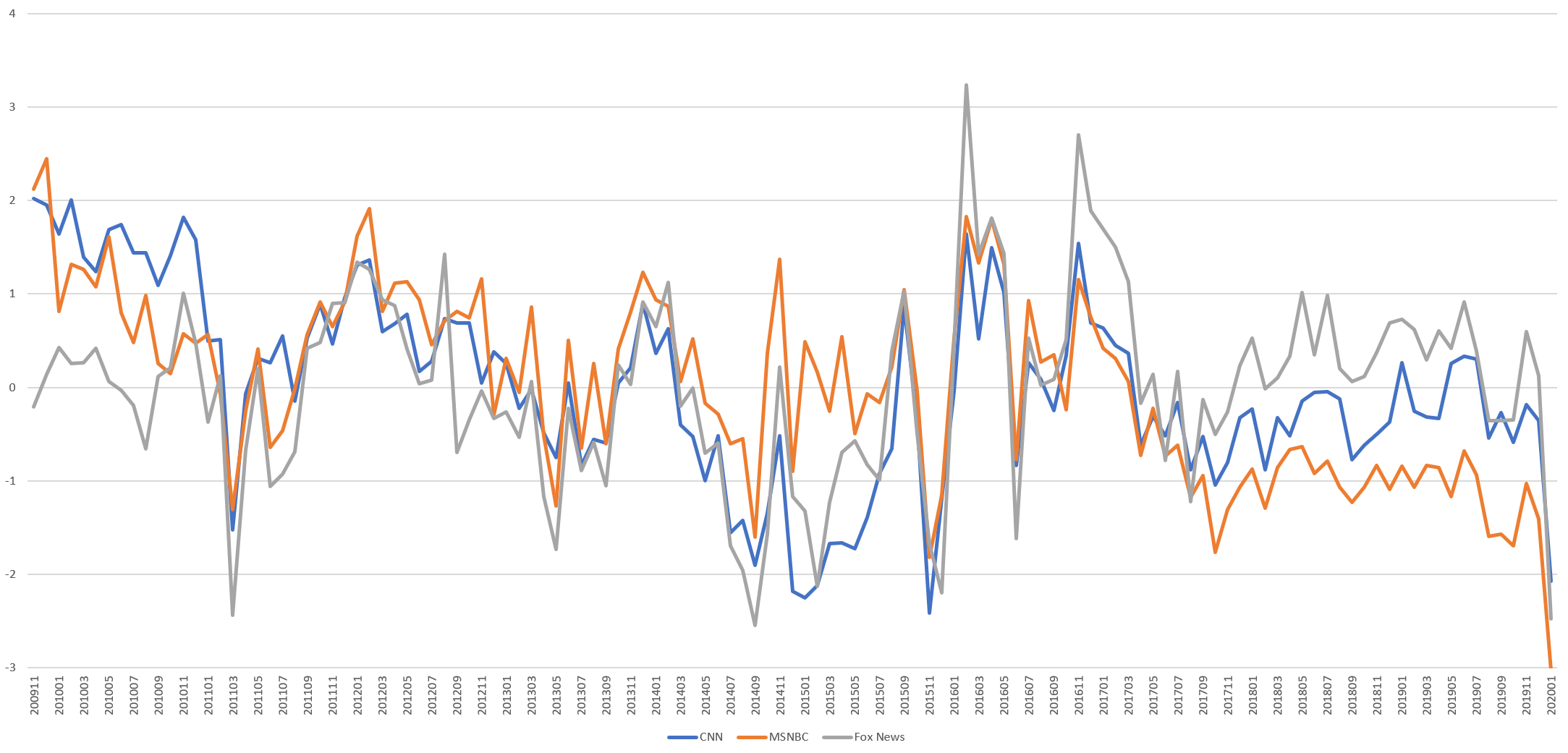# Tracing Television News Tone 2009-2020: The Trump Inflection PointHow has the tone of television news changed over the past decade? Have CNN, MSNBC and Fox News largely moved in sync or have their respective tones been highly divergent? Most importantly, has their tone changed in a measurable way since the election of Donald Trump?

It turns out we need only a single SQL query to answer all of these questions. The timeline below shows the final result, plotting the Z-score (standard deviations from mean) average monthly Linguistic Inquiry and Word Count (LIWC) tone of CNN, MSNBC and Fox News from the Internet Archive's Television News Archive.The tone of the three stations closely tracks one another until the election of Donald Trump, with a hard break since September 2017.

## TECHNICAL DETAILS

Using the same query as we used in November to compare classic and neural tone, constructing the analysis above involved just a single SQL query (swap CNN/CNNW for MSNBC/MSNBCW and FOXNEWS/FOXNEWSW to run the other two stations):

```SELECT SUBSTR(CAST(DATE AS STRING),0,6) month,
AVG(CAST(REGEXP_REPLACE(V2Tone, r',.*', "") AS FLOAT64)) gkgtone,
AVG(CAST(REGEXP_EXTRACT( GCAM, r'v19.1:([-\d\.]+)') AS FLOAT64)) anew,
AVG( (CAST(REGEXP_EXTRACT( GCAM, r'c2.131:([-\d\.]+)') AS FLOAT64) - CAST(REGEXP_EXTRACT( GCAM, r'c2.107:([-\d\.]+)') AS FLOAT64) ) / CAST(REGEXP_EXTRACT( GCAM, r'wc:([-\d\.]+)') AS FLOAT64) ) generalinquirer,
AVG( (CAST(REGEXP_EXTRACT( GCAM, r'c3.2:([-\d\.]+)') AS FLOAT64) - CAST(REGEXP_EXTRACT( GCAM, r'c3.1:([-\d\.]+)') AS FLOAT64) ) / CAST(REGEXP_EXTRACT( GCAM, r'wc:([-\d\.]+)') AS FLOAT64) ) lexicoder,
AVG( (CAST(REGEXP_EXTRACT( GCAM, r'c5.35:([-\d\.]+)') AS FLOAT64) - CAST(REGEXP_EXTRACT( GCAM, r'c5.34:([-\d\.]+)') AS FLOAT64) ) / CAST(REGEXP_EXTRACT( GCAM, r'wc:([-\d\.]+)') AS FLOAT64) ) liwc,
AVG( (CAST(REGEXP_EXTRACT( GCAM, r'c7.2:([-\d\.]+)') AS FLOAT64) - CAST(REGEXP_EXTRACT( GCAM, r'c7.1:([-\d\.]+)') AS FLOAT64) ) / CAST(REGEXP_EXTRACT( GCAM, r'wc:([-\d\.]+)') AS FLOAT64) ) opinionobserver,
AVG( (CAST(REGEXP_EXTRACT( GCAM, r'v10.1:([-\d\.]+)') AS FLOAT64) - CAST(REGEXP_EXTRACT( GCAM, r'v10.2:([-\d\.]+)') AS FLOAT64) ) ) sentiwordnet3
FROM `gdelt-bq.gdeltv2.iatvgkg` where (SourceCommonName='CNN' OR SourceCommonName='CNNW')  group by month order by month```

The query above returns several tone fields from GCAM – in this case we selected LIWC for the graph above due to its familiarity among communications scholars, though GCAM offers thousands of additional dimensions to be explored!